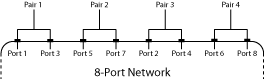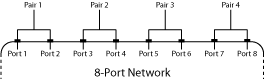Main Content

# s2smm

Convert single-ended S-parameters to mixed-mode S-parameters

## Syntax

``````[s_dd,s_dc,s_cd,s_cc] = s2smm(s_params_even,rfflag)``````
``s_mm = s2smm(s_params_odd)``

## Description

example

``````[s_dd,s_dc,s_cd,s_cc] = s2smm(s_params_even,rfflag)``` converts single-ended S-parameters to mixed-mode form.```
````s_mm = s2smm(s_params_odd)` converts single-ended odd S-parameters matrix to mixed-mode matrix. To create a mixed-mode matrix from `s_params_odd`, the single-ended input ports are paired sequentially (port1 with port 2, port 3 with port 4, etc.), and the last port is left single ended.```

## Examples

collapse all

Convert 4-port S-parameters to 2-port, mixed-mode S-Parameters.

```S = sparameters('default.s4p'); s4p = S.Parameters; [s_dd,s_dc,s_cd,s_cc] = s2smm(s4p);```

Display the 2-port mixed-mode S-Parameters at the first frequency.

`s_dd1 = s_dd(:,:,1)`
```s_dd1 = 2×2 complex -0.0124 - 0.0433i -0.5434 - 0.6872i -0.5428 - 0.6900i -0.0192 - 0.0504i ```
`s_dc1 = s_dc(:,:,1)`
```s_dc1 = 2×2 complex 0.0024 - 0.0035i -0.0005 + 0.0019i 0.0007 - 0.0012i 0.0023 - 0.0027i ```
`s_cc1 = s_cc(:,:,1)`
```s_cc1 = 2×2 complex 0.1799 - 0.1839i -0.5300 - 0.6771i -0.5314 - 0.6800i 0.1756 - 0.1910i ```
`s_cd = s_cd(:,:,1)`
```s_cd = 2×2 complex 0.0015 - 0.0029i -0.0005 + 0.0014i 0.0003 - 0.0009i 0.0019 - 0.0027i ```

## Input Arguments

collapse all

S-parameters, specified as a complex 2 N-by-2 N-by-K array, where K representing number of frequency points of 2 N-port S-Parameters. These parameters describe a device with an even number of ports.

Port order, a scalar, specified as `1`, `2`, or `3`. Port order determines how the function orders the ports:

• `rfflag = 1``s2smm` Odd-numbered ports are followed by even-numbered ports: 1,3,5,…..,2N-4,2N-2,2N.• Ports 1 and 3 become mixed-mode pair 1.

• Ports 5 and 7 become mixed-mode pair 2.

• Ports 2 and 4 become mixed-mode pair 3.

• Ports 6 and 8 become mixed-mode pair 4.

• `rfflag = 2` — Ports are paired in ascending or descending order: (1,2),…..,(2N-1,2N)• Ports 1 and 2 become mixed-mode pair 1.

• Ports 3 and 4 become mixed-mode pair 2.

• Ports 5 and 6 become mixed-mode pair 3.

• Ports 7 and 8 become mixed-mode pair 4.

• `rfflag = 3` — Half of the ports are in ascending order and half of the ports are in descending order: 1,2,…..,N,2N,2N-1,….,N+1.• Ports 1 and 2 become mixed-mode pair 1.

• Ports 3 and 4 become mixed-mode pair 2.

• Ports 8 and 7 become mixed-mode pair 3.

• Ports 6 and 5 become mixed-mode pair 4.

K (2 N+1) port single-ended S-Parameters matrix, specified as a complex (2 N+1)-by-(2 N+1)-by-K array. These parameters describe a device with an odd number of ports.

The port-ordering argument `option` is not available for (2N + 1)-by-(2N + 1)-by-K input arrays. In this case, the ports are always paired in ascending order, and the last port remains single-ended. For example, in a 7-port network:• Ports 1 and 2 become mixed-mode pair 1.

• Ports 3 and 4 become mixed-mode pair 2.

• Ports 5 and 6 become mixed-mode pair 3.

• Ports 7 remains single ended.

## Output Arguments

collapse all

Mixed-mode S-parameters, returned as complex N-by-N-by-K array, containing K matrices of differential-mode, 2N-port S-parameters (Sdd).

Mixed-mode S-parameters, returned as complex N-by-N-by-K array, containing K matrices of differential-mode, 2N-port S-parameters (Sdc).

Mixed-mode S-parameters, returned as complex N-by-N-by-K array, containing K matrices of differential-mode, 2N-port S-parameters (Scd).

Mixed-mode S-parameters, returned as complex N-by-N-by-K array, containing K matrices of differential-mode, 2N-port S-parameters (Scc).

Mixed-mode S-parameters, returned as complex N-by-N-by-K array, containing K matrices of differential-mode, 2N-port S-parameters (Smm).

`$\left[\begin{array}{lllllll}{S}_{dd,11}\hfill & \cdots \hfill & {S}_{dd,1N}\hfill & {S}_{dc,11}\hfill & \cdots \hfill & {S}_{dc,1N}\hfill & {S}_{ds,1}\hfill \\ \text{\hspace{0.17em}}⋮\hfill & \ddots \hfill & \text{\hspace{0.17em}}⋮\hfill & \text{\hspace{0.17em}}⋮\hfill & \ddots \hfill & \text{\hspace{0.17em}}⋮\hfill & \text{\hspace{0.17em}}⋮\hfill \\ {S}_{dd,N1}\hfill & \cdots \hfill & {S}_{dd,NN}\hfill & {S}_{dc,N1}\hfill & \cdots \hfill & {S}_{dc,NN}\hfill & {S}_{ds,N}\hfill \\ {S}_{cd,11}\hfill & \cdots \hfill & {S}_{cd,1N}\hfill & {S}_{cc,11}\hfill & \cdots \hfill & {S}_{cc,1N}\hfill & {S}_{cs,1}\hfill \\ \text{\hspace{0.17em}}⋮\hfill & \ddots \hfill & \text{\hspace{0.17em}}⋮\hfill & \text{\hspace{0.17em}}⋮\hfill & \ddots \hfill & \text{\hspace{0.17em}}⋮\hfill & \text{\hspace{0.17em}}⋮\hfill \\ {S}_{cd,N1}\hfill & \cdots \hfill & {S}_{cd,NN}\hfill & {S}_{cc,N1}\hfill & \cdots \hfill & {S}_{cc,NN}\hfill & {S}_{cs,N}\hfill \\ {S}_{sd,1}\hfill & \cdots \hfill & {S}_{sd,N}\hfill & {S}_{sc,1}\hfill & \cdots \hfill & {S}_{sc,N}\hfill & {S}_{ss}\hfill \end{array}\right]$`

 Granberg, T.,Handbook of Digital Techniques for High-Speed Design. Upper Saddle River, NJ: Prentice Hall, 2004.

Download ebook